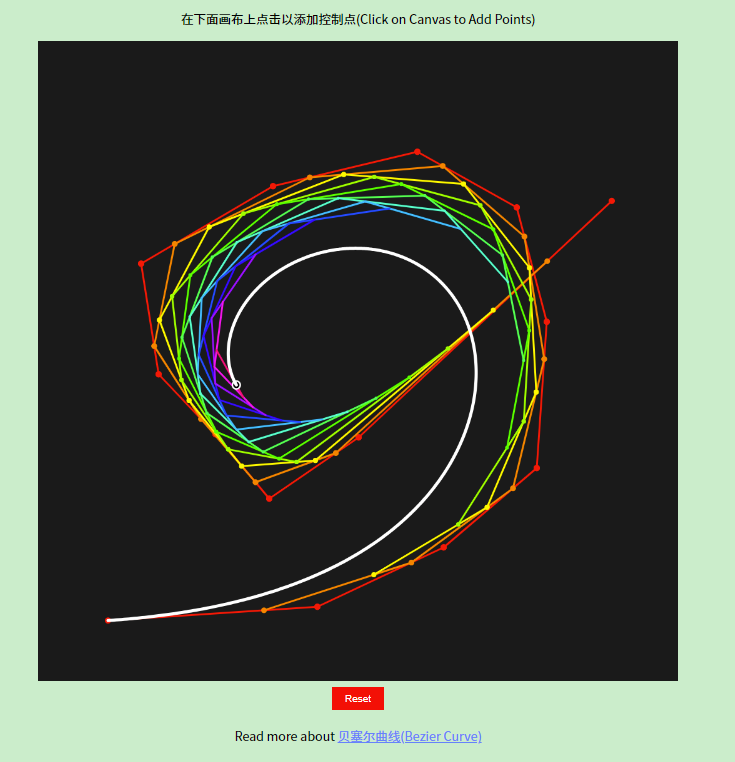By Long Luo

# 贝塞尔曲线是什么？

http://www.longluo.me/projects/bezier/# 贝塞尔曲线的数学原理

## 这一章节待完善！！！

$P_1(x_1, y_1)$$P_2(x_2, y_2)$

$\begin{cases} x = x_1 + t (x_2 - x_1) \\ y = y_1 + t (y_2 - y_1) \end{cases}$

$B(t) = P_0 + (P_1 - P_0)t= (1-t)P_0 + tP_1, t\in [0,1]$

$B(t) = \sum_{i=0}^{n} \binom{n}{i}P_i(1 - t)^{n-i}t^i = \binom{n}{0}P_0(1-t)^{n}t^0 + \binom{n}{1}P_1(1-t)^{n-1}t^1 + \cdots + \binom{n}{n-1}P_{n-1}(1-t)^{n-1}t^{n-1} + \binom{n}{n}P_{n}(1-t)^{n}t^{n}, t\in[0,1]$

$P_0^{n} = (1 - t)P_0^{n-1} + tP_1^{n-1}, t\in [0,1]$

# 贝塞尔曲线的缺点

1. 高度精确的曲线：贝塞尔曲线是由有限个控制点所定义的，当需要绘制极其精确的曲线时，可能无法满足需求；
2. 曲率变化较大的曲线：贝塞尔曲线的控制点数量决定了曲线的平滑程度。在曲率变化较大的情况下，可能需要增加更多的控制点来准确描述曲线的形状；
3. 特殊曲线类型：某些特殊曲线类型，如圆或椭圆等，可能有更适合的数学表示方法，而不是使用贝塞尔曲线。